Home | | Discrete Mathematics | Logic and Proofs

# Logic and Proofs

1 Introduction 2 Logical Connectives 3 Propositional Equivalence 4 Predicates & Quantifiers 5 Rules Of Inference 6 Introduction To Proofs Methods And Strategy

LOGIC AND PROOFS

LOGIC AND PROOFS

1 INTRODUCTION

2 LOGICAL CONNECTIVES

3 PROPOSITIONAL EQUIVALENCE

4 PREDICATES & QUANTIFIERS

5 RULES OF INFERENCE

6 INTRODUCTION TO PROOFS METHODS AND STRATEGY

1 INTRODUCTION

PROPOSITION (OR) STATEMENT:

Proposition is a declarative statement that is either true or false but not both. The truth value of proposition is true or false.

Truth table

It displays the relationship between the truth values of proposition.

Negation of a proposition

If P is a proposition, then its negation is denoted by ¬P or ~p and is defined by the following truth table.

P       ¬P

T       F

F       TEXAMPLE

P  - Ram is intelligent

¬P  -Ram is not intelligent

proposition is a declarative sentence which is either true or false but not both.

COMPOUND  PROPOSITION

It is a proposition consisting of two or more simple proposition using logical operators.

2  LOGICAL  CONNECTIVES

(1) DISJUNCTION   (OR)

The disjunction of two proposition P and Q is the propositioneadasPorQ] andP˅Qis defined by the following truth table.

P       Q       P˅Q

T       T       T

T       F       T

F       T       T

F       F       F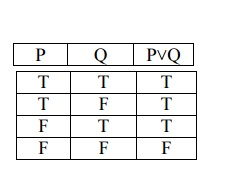(1) CONJUNCTION (AND)

If P and Q are two propositions , then the conjunction of P and Q is and Q ) and is defined by following truth table.

P       Q       P˄Q

T       T       T

T       F       F

F       T       F

F       F       FCONDITIONAL AND BI- CONDITIONAL PROPOSITION

(1) Conditional proposition

If p and q are propositions, then the implication “If p then q “ denoted by p→q , called the conditional statement of p and q , is defined by following truth table.

p       q        p→

T       T       T

T       F       F

F       T       T

F       F       TNOTE

p→q   is   false   when   p   is   true   and   q   is   false.   O

The different situations where the conditional statements applied are listed below.

(1) If p then q

(2) p only if  q

(3) q whenever  p

(4) q is necessary for  p

(5) q follows  from p

(6) q when p

(7) p is sufficient for q

(8) p implies q

Converse, contra positive and Inverse statement

If      p→q   is   a   conditional   statement,   then

(1) q→p   is   called   converse   of   p→q

(2) ¬q→¬p   is   called   contrapositive   of

(3) ¬p→¬q   is   called   inverse   of   p→q

EXAMPLE

p : Ram is a computer science student q : Ram study DBMS

p→q: If Ram is a computer science student, then the will study DBMS.

(2) Bi-conditional proposition

If p and q are proposition, then the proposition p if and only if q, denoted by  is called the bi-conditional statement and is defined by the following truth table.

p       q

T       T       T

T       F       F

F       T       F

F       F       T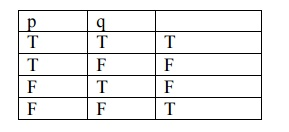NOTE

↔  is true if both p and q have same truth values. Otherwise   ↔  is false.

EXAMPLE

P:  You can take the flight

p↔q: You can take the flight if and only if buy a ticket.

Symbolize the statements using Logical Connectives

Example: 1

The automated reply can be sent when the file system is full.

P:The automated reply can be sent

Q:The file system is full

Solution:

Symbolic    form   :q→¬   p

EXAMPLE: 2

Write the symbolized form of the statement. If either Ram takes C++ or Kumar takes pascal, then Latha will take Lotus.

R:Ram takes C++

K:Kumar takes Pascal

L:Latha takes Lotus

Solution:

Symbolic           form:   (R˅K)→L

Example 3

Let p,q,r represent the following propositions,

P:It is raining

q:The sun is shining

r:There are clouds in the sky Symbolize the following statements.

(1) If it is raining, then there are clouds in the sky

(2) If it is not raining, then the sun is not shining and there are clouds in the sky.

(3) The sun is shining if and only if it is not raining.

Solution:

Symbolic form:

(1)           p →r

(2)           ¬p → (¬q˄r)

(3)           q ↔ ¬r

Example: 4

Symbolize the following statements:

(1) If the moon is out and it is not snowing, then Ram goes out for a walk.

(2) If the moon is out, then if it is not snowing,Ram goes out for a walk.

(3) It is not the case that Ram goes out for a walk if and only if it is not snowing or the moon is out.

Solution:

Let the propositions be,

P: The moon is out

Q:It is snowing

R:Ram goes out for a walk. Symbolic form:

(1)       (p˄¬q) →r

(2)       p →(¬q →r)

(3) ¬(r ↔ (¬q˅p))

Example: 5

Symbolize the following using the propositions.

P:I finish writing my computer program before lunch

q:I  shall play Tennis in afternoon.

r:The sun is shining

s:The boundary is low.

(1) If the sun is shining, I shall play tennis in the afternoon.

(2) Finishing the writing of my computer program before lunch is necessary for playing tennis in this afternoon.

(3) Low boundary and sunshine are sufficient to play Tennis in this afternoon.

Solution:

Symbolic form:

(1)  r →q

(2)  q →p

(3)  (s˄r)→q

Construction of Truth Tables

EXAMPLE: 1

Show that the truth values of the formula 𝑃˄(𝑃 → 𝑄) → 𝑄 are independent of their components.

Solution:

The truth table for the formula is,The truth values of the given formula are all true for every possible truth values of P and Q. Therefore, the truth value of the given formula is independent of their components.

Example 1. Without constructing the truth table show that p→ (q→p) ≡p(p→ q)

Solution

p→   (q→ p)p→≡(qp)

≡ p(p)

≡ p(p∨￢q)

(pp)∨￢

∨￢Tq

T.

Example 2. Prove that p→   q   is   logically   prove   that (pq)

Solution: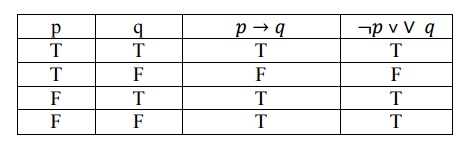EXAMPLE: 2

Write the symbolized form of the statement. If either Ram takes C++ or Kumar takes pascal, then Latha will take Lotus.

R:Ram takes C++

K:Kumar takes Pascal

L:Latha takes Lotus

Solution:

Symbolic           form:   (R˅K)→L

Tautology.

A statement that is true for all  possible  values  of  its propositional variables is called a tautology universely valid formula or a logical truth.

Example:1. Write the converse, inverse, contra positive of ‘If you work hard then you will be rewarded’

Solution:

p:      you  will be work hard.

q:       you will be rewarded.

¬p: You will not be work hard.

¬       q: You will  no tbe  rewarded.

Converse: q→ p, If you will be rewarded then you will be work hard

Contrapositive: ¬ q→ p,if You will not be rewarded then You will not be work hard.

Inverse: ¬ p→ ¬ q, if You  will not be work hard then You  will  no tbe  rewarded.

Example:2. Write the converse, inverse, contra will be rewarded’

Solution:

p:      you  will be work hard.

q:       you  will be  rewarded.

¬p: You will not be work hard.

¬ q: You     will  no tbe  rewarded.

Converse: q→ p, If you  will be  rewarded then you     will be work hard

Contrapositive: ¬ q→ p,if You  will  not be        rewarded then You will not be work hard.

Inverse: ¬ p→ ¬ q, if You  will not be work hard then You  will  no tbe   rewarded.The last column shows that S is a tautology

3 PROPOSITIONAL EQUIVALENCE:

Logical Equivalence:

Let p and q be two statements formulas, p is said to be logically equivalent to q if p & q have the same set of truth values or equivalently p & q are logically equivalent if  is tautology.

Hence,      if and only if      is a tautology.

Logical Implication or Tautological Implication

A statement formula A logically implies another, statement formula B if and only if 𝐴 → 𝐵  is a tautology.

∴ 𝐴 ⇒ 𝐵 [A logically iff 𝐴 → 𝐵 is tautology, implies B]

If 𝐴 ⇒ 𝐵 , then

A is called antecedent

B is called consequent.

Further 𝐴 ⇒ 𝐵 guarantees that B has the truth value T whenever A has the truth value T.

∴ In order to show any of the given implications, it is sufficient to show that an assignment of the truth value T to the antecedent of the given conditional leads to the truth value T for the consequent.

1. Prove without using truth table 𝑃 → 𝑄 ˄ ¬𝑄 ⇒ ¬𝑃

Proof:

Antecedent: 𝑃 → 𝑄 ˄ ¬𝑄

Consequent: ¬𝑃

Assume that, the antecedent has the truth value T.

∴ ¬𝑄 And 𝑃 → 𝑄 both are true.

⇒ Truth value of Q is F and the truth value of P is also F.

∴ Consequent ¬𝑃 is true.

∴ The truth of the antecedent implies the truth of the consequent.

∴ 𝑃 → 𝑄 ˄ ¬𝑄 ⇒ ¬𝑃

Example:1 Without constructing the truth table show that  p→ (q → p) ≡ ￢p(p→ q)

Solution

p→   (q→ p)p→≡(qp)

≡ p(p)

≡ p(p∨￢q)

(pp)∨￢

T ∨￢

T.

Example 2:Show that (p↔q)≡(pq) (pq) without constructing the truth table

Solution :

(p↔q)≡(pq) (pq)

(p↔q)≡(pq)  (qp)

(pq)  (qp)

(pq) q) ((pq) p)

(pq) (qq ) ((pp) (qp)

(pq) FF(qp)

(pq) (qp)

≡(pq)  (qp).

Consider ( ØP ÙØQ ) Ú ( ØP ÙØR ) Þ ØP ÚQ ) Ú ØP Ú R ) Þ Ø( ( P ÚQ ) ÙP Ú R ) )    ( 2 )

Using (1) and (2)

( ( P ÚQ ) ÙP ÚQ ) ÙP ÚR ) ) ÚØ( ( P ÚQ ) ÙP ÚR ) )

Þ [ ( P ÚQ ) ÙP ÚR ) ] ÚØ[ ( P ÚQ ) ÙP ÚR ) ] Þ T

Prove the following equivalences by proving the equivalences of the dual

Ø( ( ØP ÙQ ) ÚØP ÙØQ ) ) ÚP ÙQ ) ºP

Solution: It‟s dual is

Ø( ( ØP ÚQ ) ÙØP ÚØQ ) ) ÙP ÚQ ) ºP

Consider,Obtain DNF of Q  ÚP  Ù R ) ÙØ( ( P  Ú R ) ÙQ ) .

Solution:

Q  Ú ( P Ù R ) ÙØ( ( P Ú R ) Ù)

Û  ( Q  ÚP  Ù R ) ) ÙØ( ( P Ú R ) ÙQ )   ( D e m o r g a n  l a w )

Û  ( Q  ÚP  Ù R ) ) Ù( ( ØP  ÙØR ) ÚØQ )             ( D e m o r g a n  l a w )

Û  ( Q  ÙØP  ÙØR ) ) ÚQ  ÙØQ ) Ú( ( P  Ù R ) ÙØP  ÙØR ) Ú( ( P  Ù R ) ÙØ Q )

( E x t e n d e d  d i s t r i b u t e d  l a w )

Û  ( ØP  ÙQ  ÙØR ) Ú F  ÚF  Ù R  ÙØR ) ÚP  ÙØQ  Ù R )   ( N e g a t i o n  l a w )

Û  ( ØP  ÙQ  ÙØR ) ÚP  ÙØQ  Ù R )   ( N e g a t i o n  l a w )

Obtain Pcnf and Pdnf of the formula ( ØP  ÚØQ ) ® ( P  « ØQ )

Solution:PCNF: P  Ú and PDNF: ( P  Ù Q ) ÚP  Ù ØQ  ) Ú ( ØP  ÙQ )

Solution:

P ® (Ù(Q  ® ))Û ~  P Ú( P Ù( ~ Q ÚP ) )

Û  ~  P Ú( P Ù~ Q ) Ú( P Ù)

Û ( ~  P ÙT ) ÚP Ù ~ Q ) ÚP ÙP )

Û ( ~  P ÙQ Ú ~ Q ) ÚP Ù ~ Q ) ) ÚP ÙQ Ú ~ Q ) )

4 PREDICATES & QUANTIFIERS:

Quantifiers.

Universal Quantifiers:

The universal Quantification of P(x) is the proposition.”P(x) is true for all values of x in the universe of discourse”.

The notation ¥x P(x) denotes the universal quantification of P(x).here ¥ is called the universal quantifier.

Existential Quantifier:

The existential Quantification of P(x) is the proposition.” There exists an element x in the universe of discourse such that P(x) is true”.

We use the notation Ǝx P(x) for the existential quantification of p(x).here Ǝ is called the existential quantifier.

Normal Forms:

DNF:

A formula which  is equivalent to a given formula and which consists o sum of elementary products is called  a disjunctive normal form of the given formula

PDNF: a formula which is equivalent to a given formula which is consists of sum its minterms is called PDNF.

PCNF: a formula which is equivalent to a given formula which consists of product of maxterms is called PCNF.

Obtain PCNF of (￢p→ r) ∧(q↔ p). and hence obtain its PDNF.

Solution:

PCNF: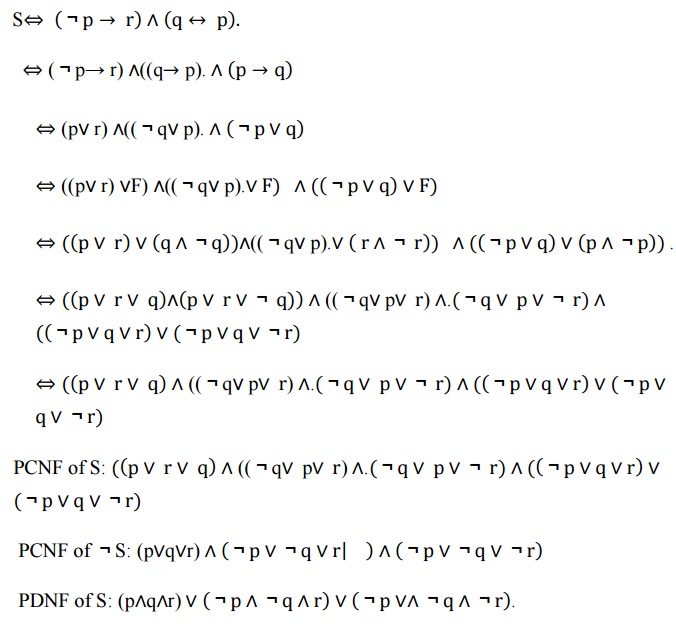S  p  r  q  p .

⇔ (p→  r)((qp). ∧ p →q

(pr) ((qp).  p ∨q

((pr) F) ((qp).∨F) ∧( p ∨q ∨F)

( p ∨ r ∨ q ∧q )((qp).∨ r ∧ r ) ∧( p ∨q ∨ p ∧p ) .

( p ∨ r ∨ q p ∨ r ∨ q) ∧((qp∨ r) ∧.(q ∨ p ∨ r) ∧

p ∨q ∨r ∨(p ∨q ∨r)

⇔ ( p ∨ r ∨ q ∧((qp∨ r) ∧.(q ∨ p ∨ r) ∧( p ∨q ∨r ∨(p ∨ q ∨r)

PCNF of S: ( p  r  q ((q p r.(q  p  r) ∧( p ∨q ∨r  (p ∨q ∨r)

PCNF of S: (pqr)  p q ∨r       ∧ p ∨q ∨r

PDNF of S: (pqr)  p q ∧r  p ∨∧q r .

5 RULES OF INFERENCE

EXAMPLE:1 Verify that validating of the following inference. If one person is more successful than another, then he has worked harder to deserve success. Ram has not worked harder than Siva. Therefore, Ram is not more successful than Siva.

Solution:

Let the universe consists of all persons.

Let S(x,y): x is more successful than y.

H(x,y): x has worked harder than y to deserve success.

a: Ram

b: Siva

Then, given set of premises are

1) (x) (y) [S(x,y) ®H(x,y)]

2)    H(a,b)

3)    Conslution is S(a,b).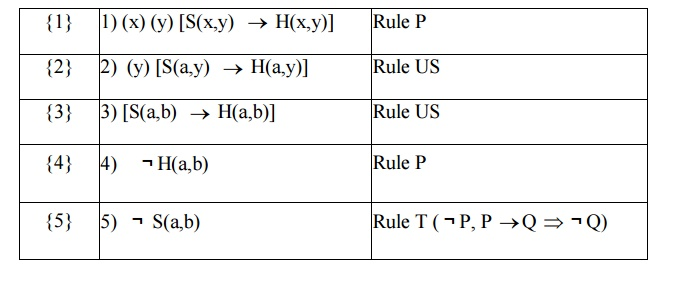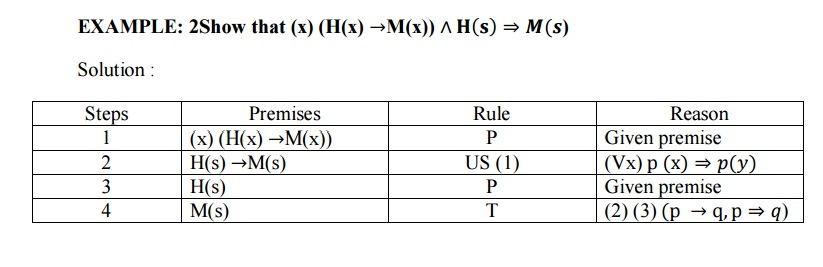EXAMPLE: 3  Show that ¬ p(a,b) follows logically from (x) (y) (p(x,y)

→w(x,y)) 𝒂𝒏𝒅 ¬𝒘 𝒂, 𝒃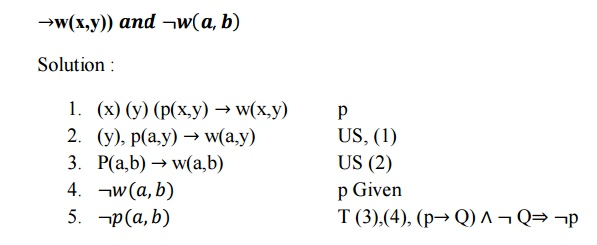EXAMPLE:4.

Symbolise: For every x, these exixts a y such that x2+y2 ≥100

Solution :

"x ) (  ) (x2+y2 ≥   100)

Example:Let p, q, r be the following statements:

p: I will study discrete mathematics q: I will watch T.V.

r: I am in a good mood.

Write the following statements in terms of p, q, r and logical connectives. (1) If I do not study and I watch T.V., then I am in good mood.

(2)If I am in good mood, then I will study or I will watch T.V.

(3)If I am not in good mood, then I will not watch T.V. or I will study.

(1 ) ( Øp Ùq ) ® r

( 2 ) r  ® p Úq )

( 3 ) Ør ® Øq Ú p )

6 Introduction to proofs & statergy

Method of proofs :

Trival proof:

In an implication p ® q , if we can establish that q is true , then regardless of the truth value of p, the implication p ® q So the construction of a trivial proof of p ® q needs to show that the truth value of q is true.

Vacous proof:

If the hypothesis p of an implication p ® q is false , then p ® q is true for any proposition q.

Prove that Rt(2) is irrational.

Solution :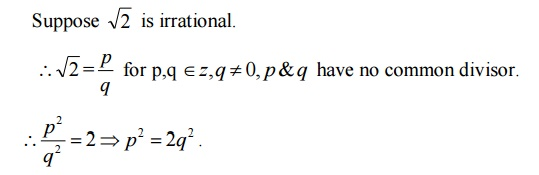Since  p2  is  an even integer, p is an even integer.

\ p= 2m for some integer m.

\  (2m)2  =2q2 Þ q2  =2m2

Since q 2  is  an even integer, q is an even integer.

\ q= 2k f or some integer k.

Thus p & q are even . Hence they have a common factor 2. Which is a contradiction to our assumption.

\RT(2) is irrational.

Study Material, Lecturing Notes, Assignment, Reference, Wiki description explanation, brief detail
Mathematics (maths) : Discrete Mathematics : Logic and Proofs : Logic and Proofs |

Related Topics